# Ant Nests

Locations of ant nests with marks denoting two types of species

## Details

Locations of ant nests in the observation region Rectangle[{-7, -1}, {787, 673}] in feet, annotated with species Cataglyphis/Messor.

## Examples

### Basic Examples (3)

Retrieve the data:

 In:=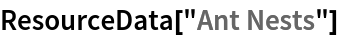Out=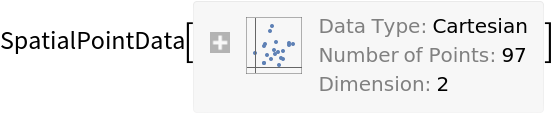Summary of the spatial point data:

 In:=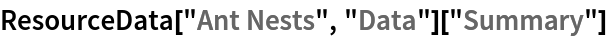Out=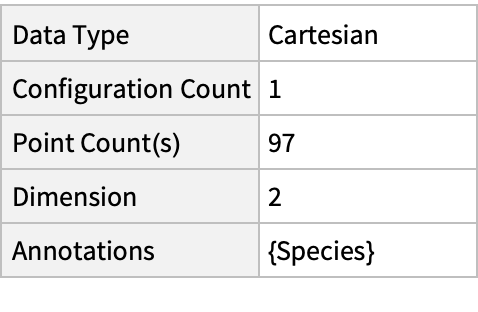Plot the points:

 In:=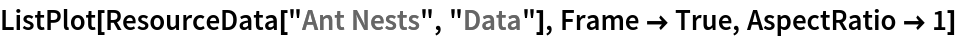Out=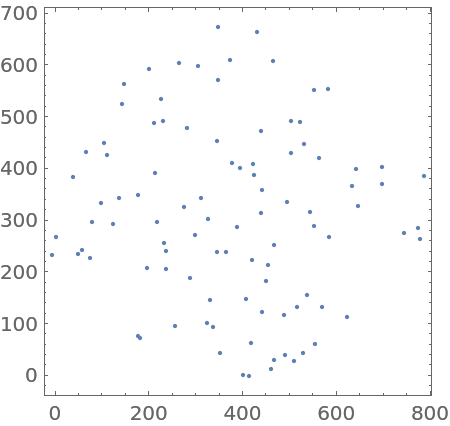### Scope & Additional Elements (1)

Select subsets based on the species type:

 In:=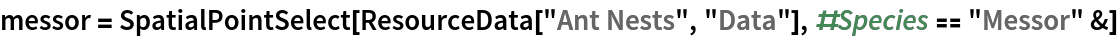Out=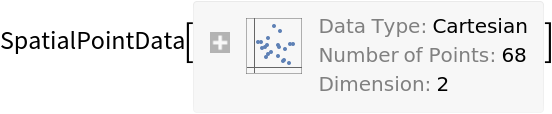In:=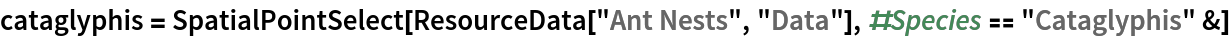Out=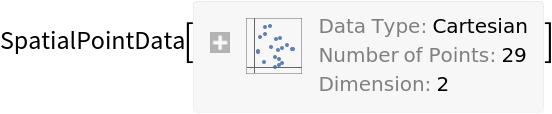### Visualizations (1)

Plot locations with information about species:

 In:=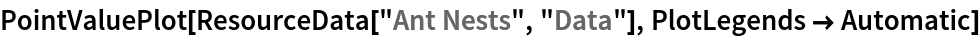Out=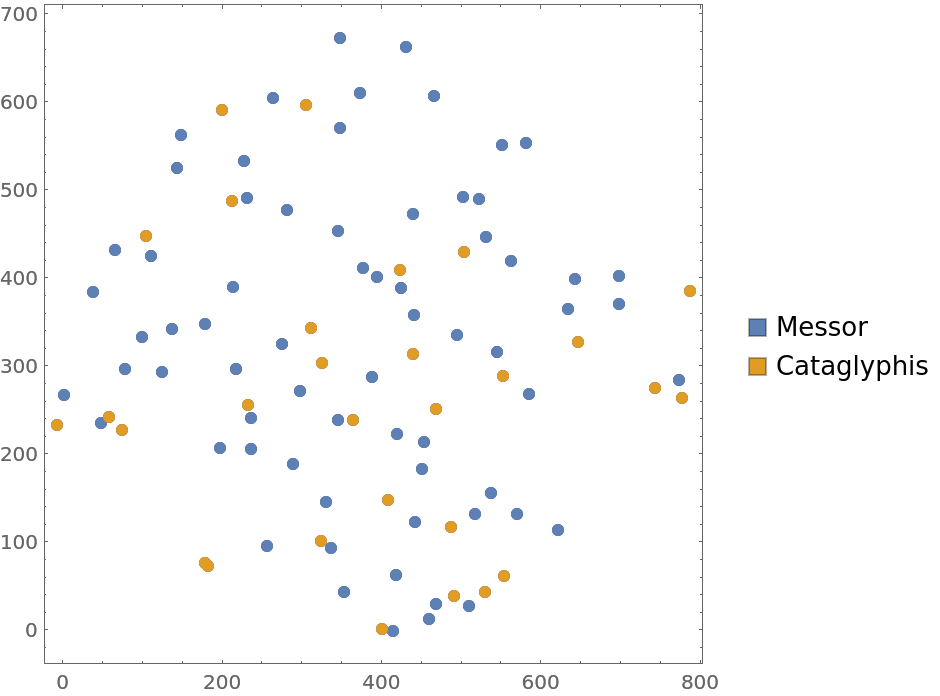### Analysis (4)

Test for complete spacial randomness:

 In:=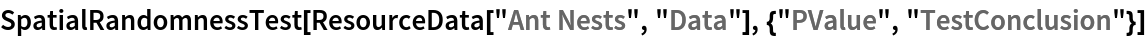Out=Fit a hard core point process to data:

 In:=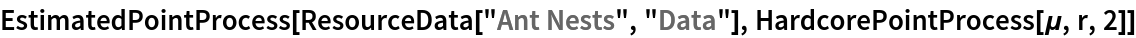Out=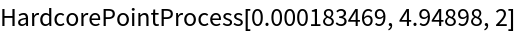Compute probability of finding a point within given radius of an existing point - NearestNeighborG is the CDF of the nearest neighbor distribution:

 In:=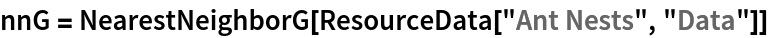Out=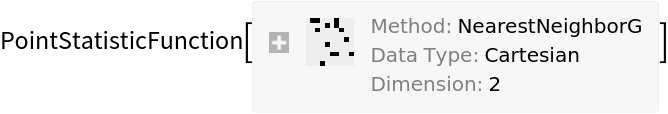In:=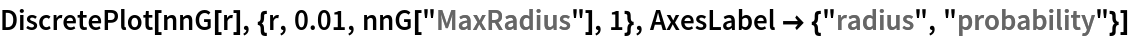Out=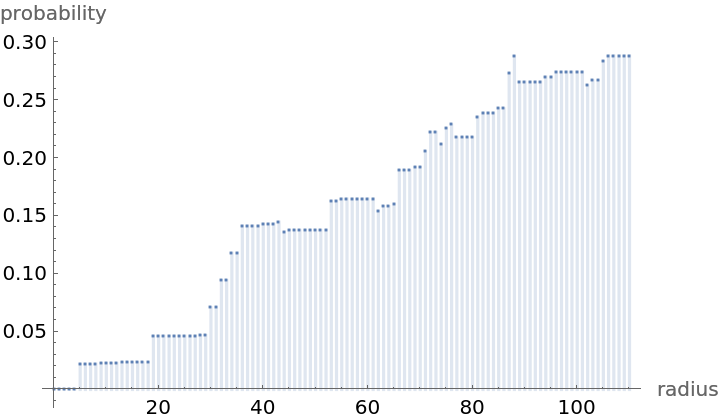Mean distance between a typical point and its nearest neighbor (for positive support distribution can be approximated via a Riemann sum of 1-CDF):

 In:=In:=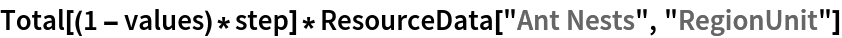Out=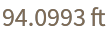Gosia Konwerska, "Ant Nests" from the Wolfram Data Repository (2021)